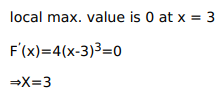# Find the point of local maxima or local minima or local minima and the corresponding local

Question:

Find the point of local maxima or local minima or local minima and the corresponding local maximum and minimum values of each of the following functions:

$f(x)=(x-3)^{4}$

Solution:$\square$ local max. Vaue is $0 .$